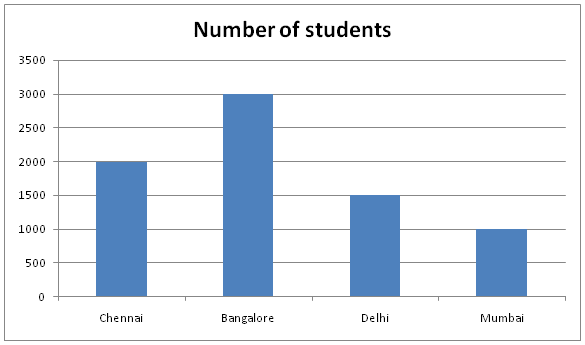# IBPS PO Prelims Quantitative Aptitude (Day-01)

Dear Aspirants, Our IBPS Guide team is providing new series of Quantitative Aptitude Questions for IBPS PO Prelims 2020 so the aspirants can practice it on a daily basis. These questions are framed by our skilled experts after understanding your needs thoroughly. Aspirants can practice these new series questions daily to familiarize with the exact exam pattern and make your preparation effective.

Start Quiz

Application Sums

1) Pipe A and pipe B fill the tank in 15 hours and 10 hours respectively. If both pipes are opened simultaneously and after 4 hours Pipe B is closed, then how much time will Pipe A take to fill the remaining tank?

A) 6 hours

B) 8 hours

C) 5 hours

D) 4 hours

E) None of these

2) Ram drive the car at the speed of 40kmph from his home to office and returning through the same route and he got stuck in traffic and take 45 minutes more to reach home, also he drive only at 32kmph. Find the distance between home to office.

A) 100km

B) 120km

C) 80km

D) 110km

E) None of these

3) The ratio of the radius of Cone to that of Cylinder is 2:3 and the ratio of the height of cone to that of cylinder is 3:4. If the length of the cone is half of the height of cylinder, then find the ratio of Curved surface area of Cylinder to that of Cone.

A) 6:1

B) 3:4

C) 5:2

D) 6:5

E) Cannot be determined

4) A and B started the business with the investment of Rs.100 and Rs.50 and after 4 months C joined with the investment of Rs.150 and B added Rs.50 with his initial investment. If the profit share of C is Rs.3600, then find the total profit of the business.

A) Rs.10200

B) Rs.8000

C) Rs.10000

D) Rs.12000

E) None of these

5) A train crosses the 200m long tunnel in 54 seconds and also crosses the 100m long bridge in 36 seconds, then find the speed of the train.

A) 20kmph

B) 40kmph

C) 60kmph

D) 80kmph

E) Cannot be determined

Data Interpretation

Direction (6 – 8): Study the following information carefully and answer the given questions:

The given bar graph shows the number of students appearing the exam from 4 different cities.6) The number of the students appearing the exam from Chennai is what percent of the number of students appearing for exam from Delhi and Mumbai together?

A) 40%

B) 60%

C) 80%

D) 90%

E) None of these

7) If the number of passed students from Bangalore is 45%, then find the number of Bangalore students who failed in the examination.

A) 1540

B) 1650

C) 1760

D) 1830

E) None of these

8) What is the average number of students appearing for exam from all the cities together?

A) 1875

B) 1925

C) 1950

D) 1890

E) None of these

Data Sufficiency

Direction (9 – 10): The following questions are accompanied by two statements I and II. You have to determine which statement is/are sufficient to answer the question.

9) What is the three digit number?

Statement I: The three digit number is exact divisible by 7.

Statement II: Sum of the first and third digit is 7.

A) Only I is sufficient to answer the question.

B) Only II is sufficient to answer the question.

C) Either I or II sufficient is sufficient to answer the question.

D) Both I and II are necessary to the answer the question

E) The question can’t be answered even with all I and II

10) What is the profit earned by selling the shirt for Rs.200?

Statement I: 25% of the profit is earned by selling each shirt.

Statement II: The cost price of 5 shirts is equal to the selling price of 4 shirts.

A) Only I is sufficient to answer the question.

B) Only II is sufficient to answer the question.

C) Either I or II sufficient is sufficient to answer the question.

D) Both I and II are necessary to the answer the question

E) The question can’t be answered even with all I and II

Directions (1-5) :

A+B=1/15+1/10= (2+3)/30=5/30=1/6

(A+B) fill the tank in 4 hours=4/6=2/3

Remaining work=1-2/3=1/3

A= (1/3)*15=5 hours

Let required distance be x km.

x/32-x/40=45/60

(5x-4x)/160=3/4

x=120km

Required ratio=2*22/7*3x*4y:22/7*2x*2y

=6:1

Profit ratio=100*12:(50*4+100*8):150*8

= 1200 : 1000 : 1200

=6:5:6

Total profit=17/6*3600=Rs. 10200

Let Length of the train=x

And Speed of the train=y

x+200=y*5/18*54

x+200=15y

x+100=y*5/18*36

x+100=10y

15y-200+100=10y

5y=100

y=20kmph

Directions (6-8) :

Required percentage= [2000/(1500+1000)]*100

=80%

Required Average=(2000+3000+1500+1000)/4=1875

Directions (9-10) :

From statement I,

The three digit number is exact divisible by 7.

So, Statement I alone is not sufficient to the answer the question.

From statement II,

Sum of the first and third digit is 7.

So, Statement II alone is not sufficient to the answer the question.

From Statement I,

Required Profit=25/125*200=Rs. 40

So, Statement I alone is sufficient to the answer the question.

From statement II,

Profit %= (5-4)/4*100=25%

Then Profit= (25/125) *200= Rs. 40

So, Statement II alone is sufficient to the answer the question.